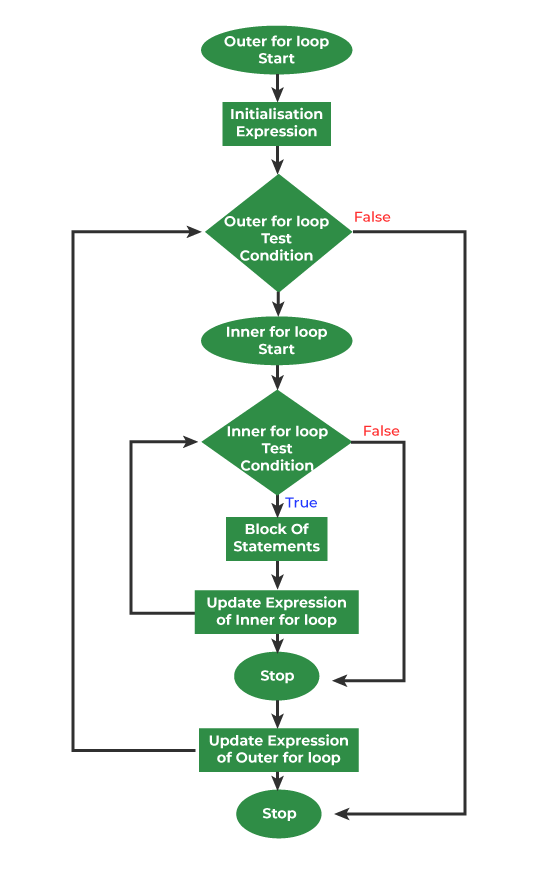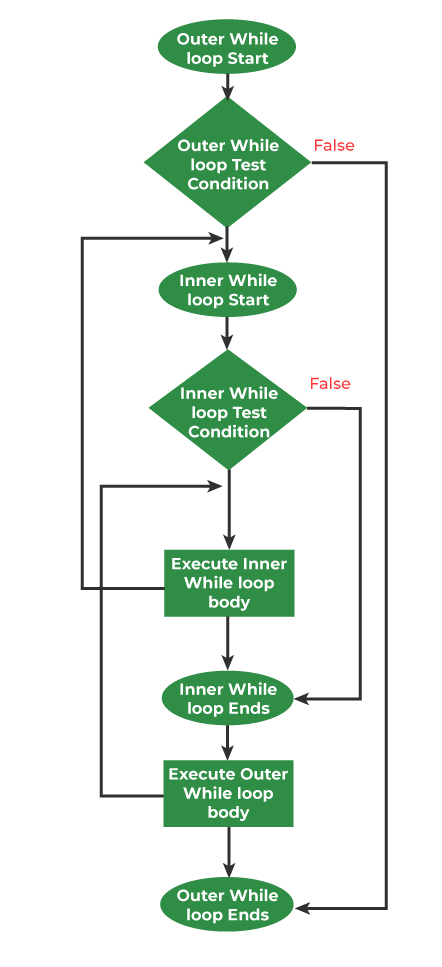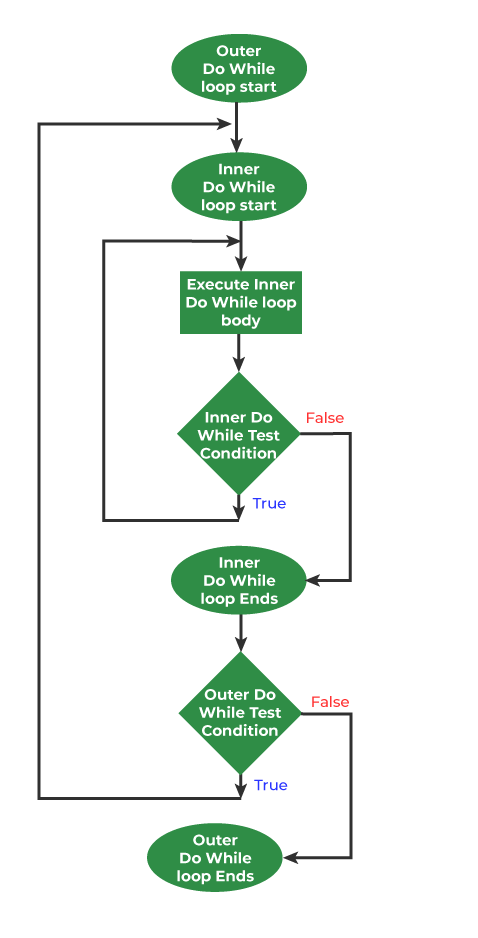Open in App
Not now

# Nested Loops in C with Examples

• Difficulty Level : Medium
• Last Updated : 25 Oct, 2022

A nested loop means a loop statement inside another loop statement. That is why nested loops are also called “loop inside loops“. We can define any number of loops inside another loop.

## 1. Nested for Loop

Nested for loop refers to any type of loop that is defined inside a ‘for’ loop. Below is the equivalent flow diagram for nested ‘for’ loops:Nested for loop in C

Syntax:

```for ( initialization; condition; increment ) {

for ( initialization; condition; increment ) {

// statement of inside loop
}

// statement of outer loop
}```

Example: Below program uses a nested for loop to print a 3D matrix of 2x3x2.

## C

 `// C program to print elements of Three-Dimensional Array``// with the help of nested for loop``#include `` ` `int` `main()``{``    ``// initializing the 3-D array``    ``int` `arr``        ``= { { { 0, 6 }, { 1, 7 }, { 2, 8 } },``            ``{ { 3, 9 }, { 4, 10 }, { 5, 11 } } };`` ` `    ``// Printing values of 3-D array``    ``for` `(``int` `i = 0; i < 2; ++i) {``        ``for` `(``int` `j = 0; j < 3; ++j) {``            ``for` `(``int` `k = 0; k < 2; ++k) {``                ``printf``(``"Element at arr[%i][%i][%i] = %d\n"``,``                       ``i, j, k, arr[i][j][k]);``            ``}``        ``}``    ``}``    ``return` `0;``}`

Output

```Element at arr = 0
Element at arr = 6
Element at arr = 1
Element at arr = 7
Element at arr = 2
Element at arr = 8
Element at arr = 3
Element at arr = 9
Element at arr = 4
Element at arr = 10
Element at arr = 5
Element at arr = 11```

## 2. Nested while Loop

A nested while loop refers to any type of loop that is defined inside a ‘while’ loop. Below is the equivalent flow diagram for nested ‘while’ loops:Nested while loop in C

Syntax:

```while(condition) {

while(condition) {

// statement of inside loop
}

// statement of outer loop
}```

Example: Print Pattern using nested while loops

## C

 `// C program to print pattern using nested while loops``#include `` ` `int` `main()``{``    ``int` `end = 5;`` ` `    ``printf``(``"Pattern Printing using Nested While loop"``);`` ` `    ``int` `i = 1;`` ` `    ``while` `(i <= end) {``        ``printf``(``"\n"``);``        ``int` `j = 1;``        ``while` `(j <= i) {``            ``printf``(``"%d "``, j);``            ``j = j + 1;``        ``}``        ``i = i + 1;``    ``}``    ``return` `0;``}`

Output

```Pattern Printing using Nested While loop
1
1 2
1 2 3
1 2 3 4
1 2 3 4 5 ```

## 3. Nested do-while Loop

A nested do-while loop refers to any type of loop that is defined inside a do-while loop. Below is the equivalent flow diagram for nested ‘do-while’ loops:do while loop in C

Syntax:

```do{

do{

// statement of inside loop
}while(condition);

// statement of outer loop
}while(condition);```

Note: There is no rule that a loop must be nested inside its own type. In fact, there can be any type of loop nested inside any type and to any level.

Syntax:

```do{

while(condition) {

for ( initialization; condition; increment ) {

// statement of inside for loop
}

// statement of inside while loop
}

// statement of outer do-while loop
}while(condition);```

Example: Below program uses a nested for loop to print all prime factors of a number.

## C

 `// C Program to print all prime factors``// of a number using nested loop`` ` `#include ``#include `` ` `// A function to print all prime factors of a given number n``void` `primeFactors(``int` `n)``{``    ``// Print the number of 2s that divide n``    ``while` `(n % 2 == 0) {``        ``printf``(``"%d "``, 2);``        ``n = n / 2;``    ``}`` ` `    ``// n must be odd at this point. So we can skip``    ``// one element (Note i = i +2)``    ``for` `(``int` `i = 3; i <= ``sqrt``(n); i = i + 2) {``        ``// While i divides n, print i and divide n``        ``while` `(n % i == 0) {``            ``printf``(``"%d "``, i);``            ``n = n / i;``        ``}``    ``}`` ` `    ``// This condition is to handle the case when n``    ``// is a prime number greater than 2``    ``if` `(n > 2)``        ``printf``(``"%d "``, n);``}`` ` `/* Driver program to test above function */``int` `main()``{``    ``int` `n = 315;``    ``primeFactors(n);``    ``return` `0;``}`

Output:

`3 3 5 7`

## Break Inside Nested Loops

Whenever we use a break statement inside the nested loops it breaks the innermost loop and program control is inside the outer loop.

Example:

## C

 `// C program to show working of break statement``// inside nested for loops``#include `` ` `int` `main()``{`` ` `    ``int` `i = 0;``    ``for` `(``int` `i = 0; i < 5; i++) {``        ``for` `(``int` `j = 0; j < 3; j++) {``            ``// This inner loop will break when i==3``            ``if` `(i == 3) {``                ``break``;``            ``}``            ``printf``(``"* "``);``        ``}``        ``printf``(``"\n"``);``    ``}``    ``return` `0;``}`

Output

```* * *
* * *
* * *

* * * ```

In the above program, the first loop will iterate from 0 to 5 but here if i will be equal to 3 it will break and will not print the * as shown in the output.

## Continue Inside Nested loops

Whenever we use a continue statement inside the nested loops it skips the iteration of the innermost loop only. The outer loop remains unaffected.

Example:

## C

 `// C program to show working of continue statement``// inside nested for loops``#include `` ` `int` `main()``{`` ` `    ``int` `i = 0;``    ``for` `(``int` `i = 0; i < 5; i++) {``        ``for` `(``int` `j = 0; j < 3; j++) {``            ``// This inner loop will skip when j==2``            ``if` `(j==2) {``                ``continue``;``            ``}``            ``printf``(``"%d "``,j);``        ``}``        ``printf``(``"\n"``);``    ``}``    ``return` `0;``}`

In the above program, the inner loop will be skipped when j will be equal to 2. The outer loop will remain unaffected.

My Personal Notes arrow_drop_up